# The figure below shows two masses m,=4.5 kg and m2=4.1 kg which are connected by a...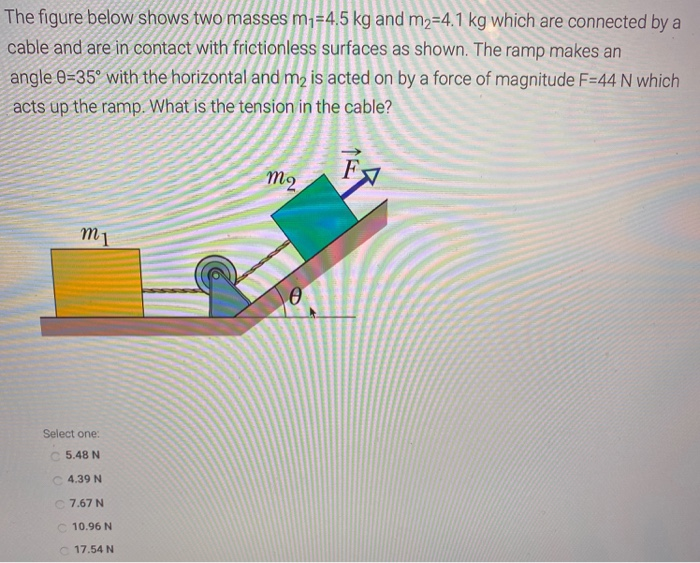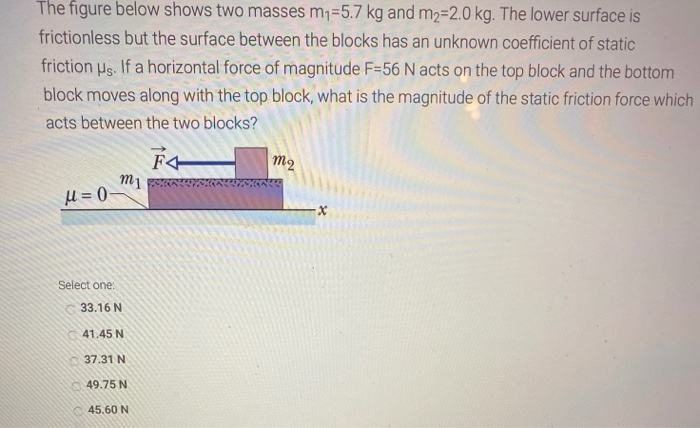The figure below shows two masses m,=4.5 kg and m2=4.1 kg which are connected by a cable and are in contact with frictionless surfaces as shown. The ramp makes an angle 0=35° with the horizontal and m is acted on by a force of magnitude F=44 N which acts up the ramp. What is the tension in the cable? m2 m 1 e Select one: 5.48 N C4.39 N 7.67 N 10.96 N C17.54 N
The figure below shows two masses m1=5.7 kg and m2=2.0 kg. The lower surface is frictionless but the surface between the blocks has an unknown coefficient of static friction us. If a horizontal force of magnitude F=56 N acts on the top block and the bottom block moves along with the top block, what is the magnitude of the static friction force which acts between the two blocks? F mi u=0 m2 Select one: 33.16 N 41.45 N 37.31 N 49.75 N 45.60 N

Question 1)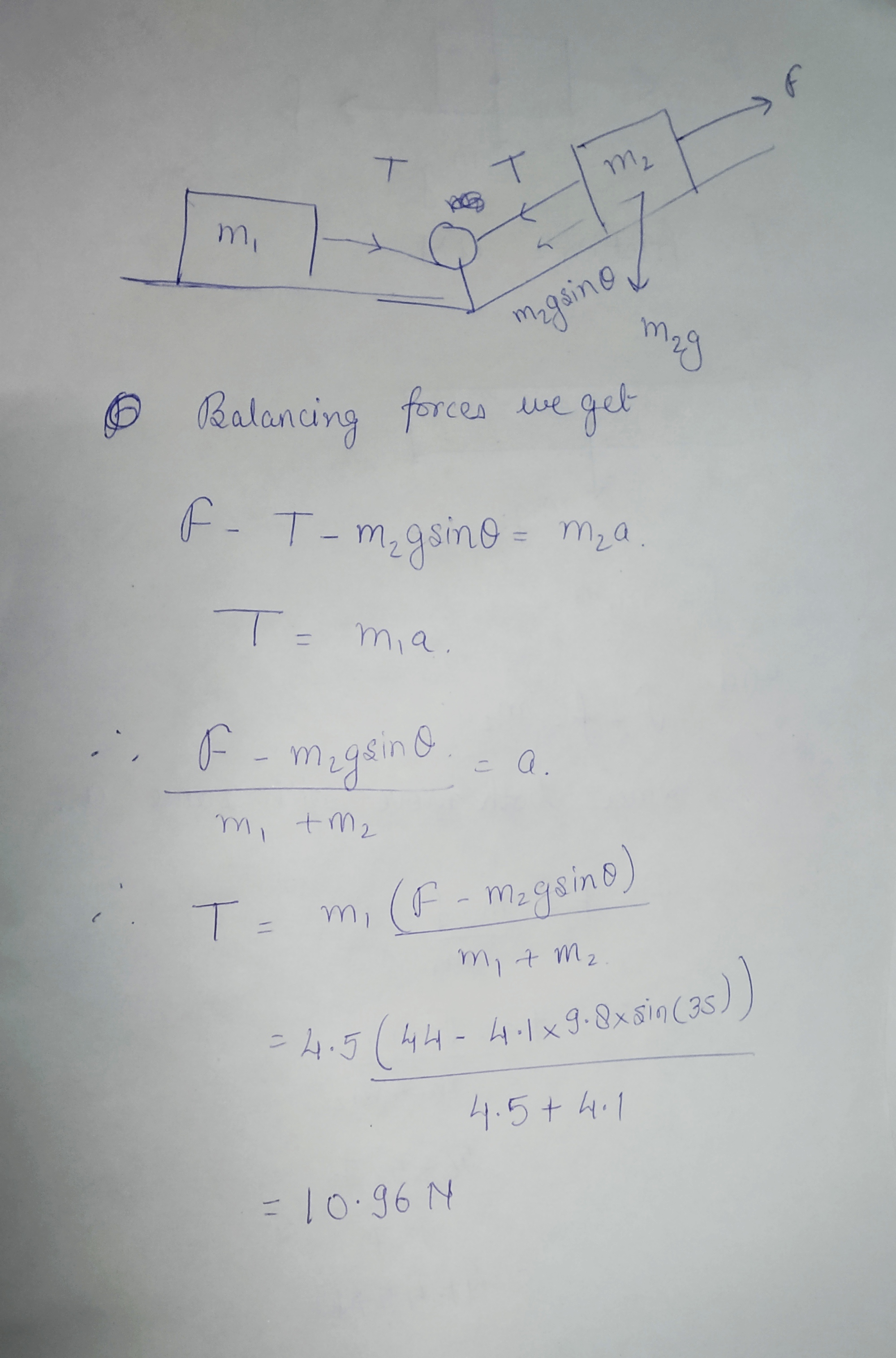Question 2)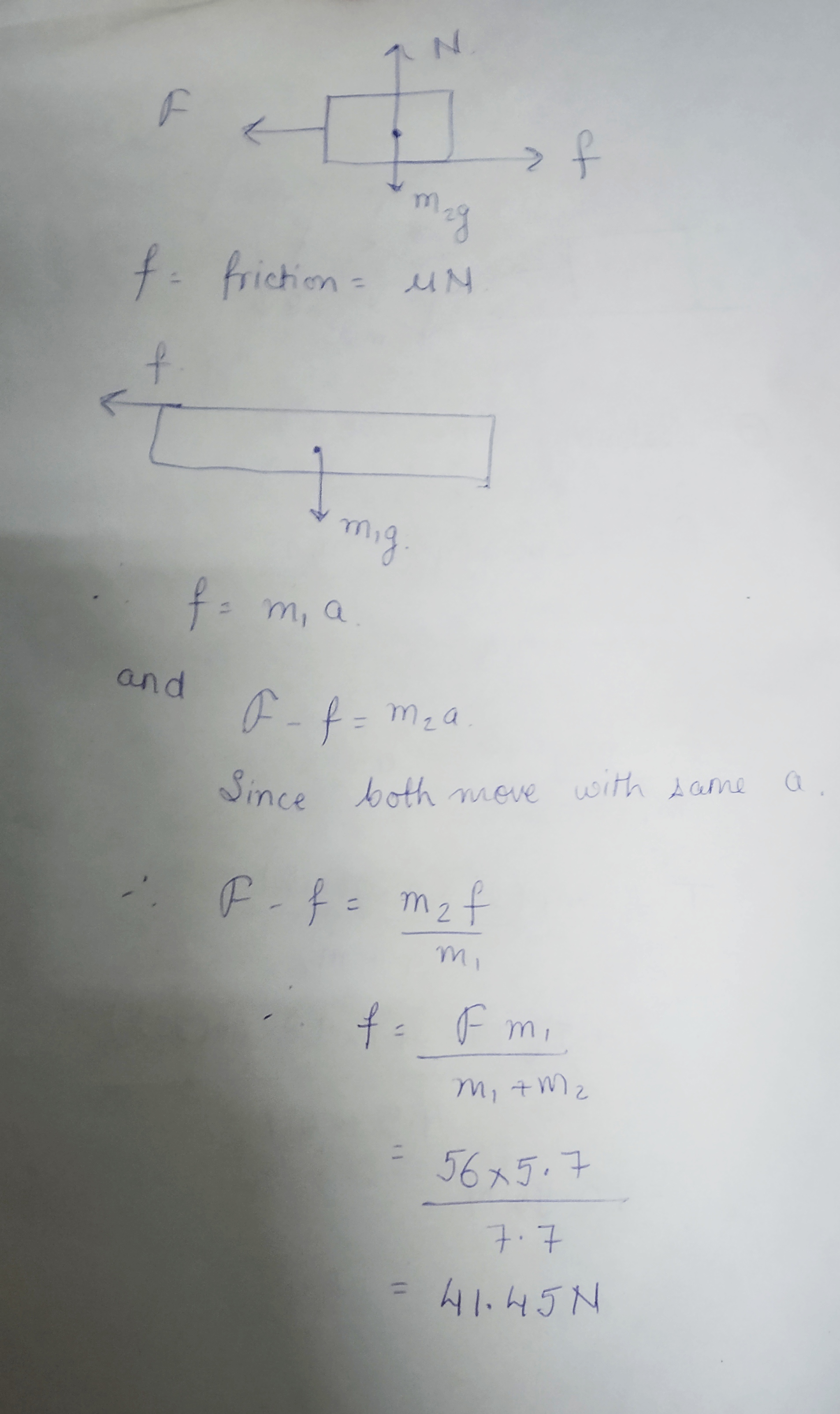##### Add Answer of: The figure below shows two masses m,=4.5 kg and m2=4.1 kg which are connected by a...
Similar Homework Help Questions
• ### The figure below shows two masses m,=5.7 kg and m2=2.0 kg. The lower surface is frictionless...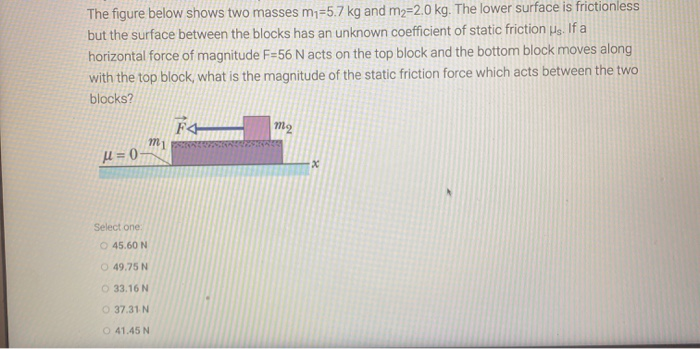The figure below shows two masses m,=5.7 kg and m2=2.0 kg. The lower surface is frictionless but the surface between the blocks has an unknown coefficient of static friction us. If a horizontal force of magnitude F=56 N acts on the top block and the bottom block moves along with the top block, what is the magnitude of the static friction force which acts between the two blocks? F4 mi m2 U=0 x Select one 45.60 N 49.75 N 33.16...

• ### The figure below shows two masses m1=4.1 kg and m2=1.9 kg. The lower surface is frictionless...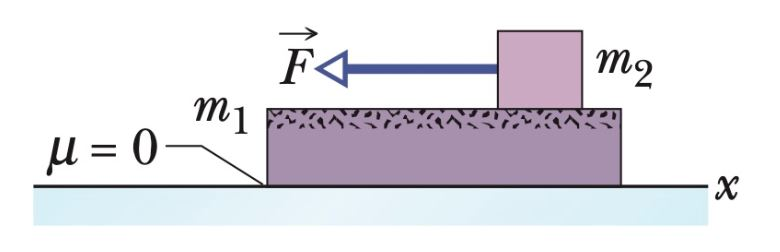The figure below shows two masses m1=4.1 kg and m2=1.9 kg. The lower surface is frictionless but the surface between the blocks has an unknown coefficient of static friction µs. If a horizontal force of magnitude F=44 N acts on the top block and the bottom block moves along with the top block, what is the magnitude of the static friction force which acts between the two blocks? X ( = ni Іш ՇՂԱ FA

• ### The figure below shows two masses m1=4.5 kg and m2=4.1 kg which are connected by a...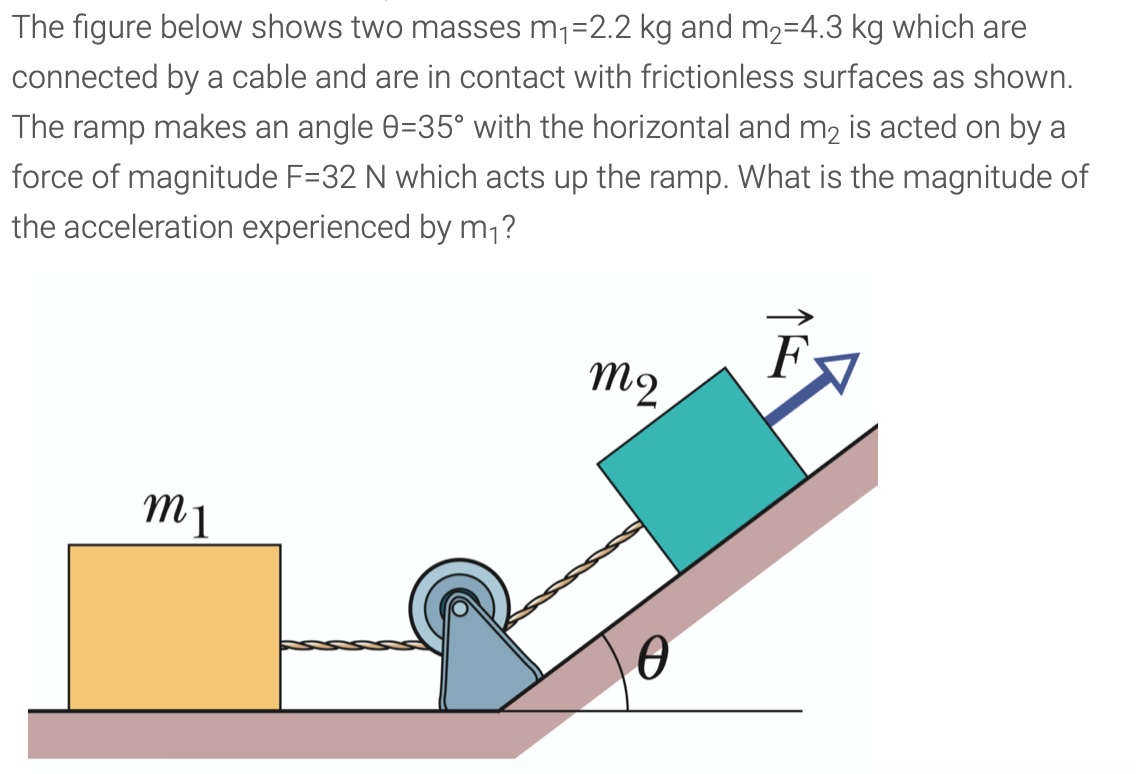The figure below shows two masses m1=4.5 kg and m2=4.1 kg which are connected by a cable and are in contact with frictionless surfaces as shown. The ramp makes an angle θ=35° with the horizontal and m2 is acted on by a force of magnitude F=44 N which acts up the ramp. What is the tension in the cable? (ANSWER ABOVE QUESTION, but use figure below) The figure below shows two masses m1=2.2 kg and m2=4.3 kg which are connected...

• ### The figure below shows two masses m,=5.6 kg and m2=1.7 kg. The lower surface is frictionless...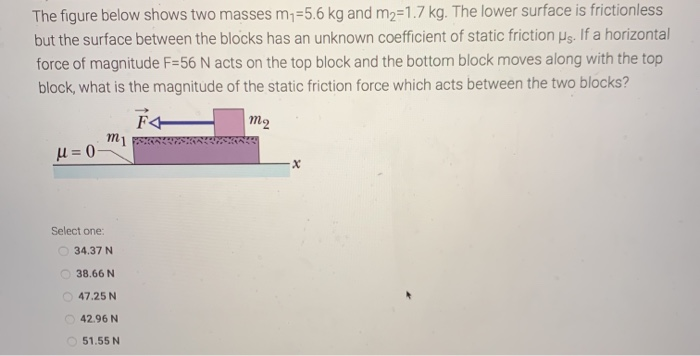The figure below shows two masses m,=5.6 kg and m2=1.7 kg. The lower surface is frictionless but the surface between the blocks has an unknown coefficient of static friction us. If a horizontal force of magnitude F=56 N acts on the top block and the bottom block moves along with the top block, what is the magnitude of the static friction force which acts between the two blocks? A mi U = 0 m2 X Select one: 34.37 N 38.66...

• ### The figure below shows two masses my=5.9 kg and m2=3.6 kg. The lower surface is frictionless...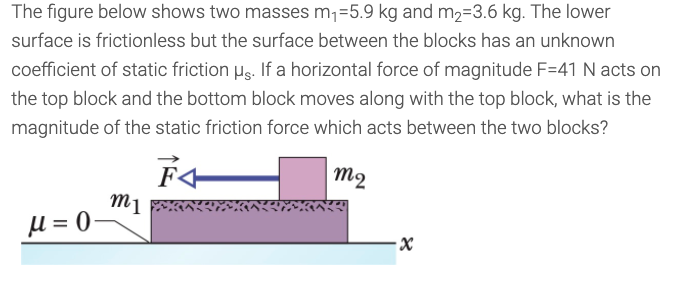The figure below shows two masses my=5.9 kg and m2=3.6 kg. The lower surface is frictionless but the surface between the blocks has an unknown coefficient of static friction us. If a horizontal force of magnitude F=41 N acts on the top block and the bottom block moves along with the top block, what is the magnitude of the static friction force which acts between the two blocks? m2 mi U = 0 х

• ### The figure below shows two masses my=5.9 kg and m2=3.6 kg. The lower surface is frictionless...The figure below shows two masses my=5.9 kg and m2=3.6 kg. The lower surface is frictionless but the surface between the blocks has an unknown coefficient of static friction us. If a horizontal force of magnitude F=41 N acts on the top block and the bottom block moves along with the top block, what is the magnitude of the static friction force which acts between the two blocks? m2 mi U = 0 х

• ### The figure below shows two masses m1=4.5 kg and m2=4.1 kg which are connected by a...

The figure below shows two masses m1=4.5 kg and m2=4.1 kg which are connected by a cable and are in contact with frictionless surfaces as shown. The ramp makes an angle θ=35° with the horizontal and m2 is acted on by a force of magnitude F=44 N which acts up the ramp. What is the tension in the cable?

• ### The figure below shows two masses m1=5.9 kg and m2=3.3 kg. The lower surface is frictionless...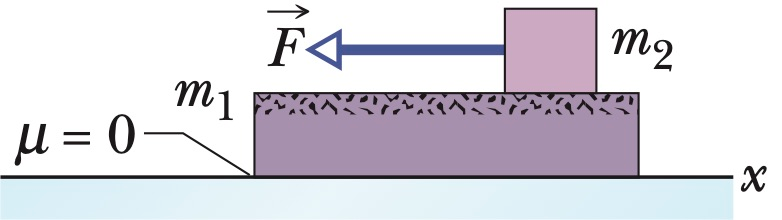The figure below shows two masses m1=5.9 kg and m2=3.3 kg. The lower surface is frictionless but the surface between the blocks has an unknown coefficient of static friction µs. If a horizontal force of magnitude F=57 N acts on the top block and the bottom block moves along with the top block, what is the magnitude of the static friction force which acts between the two blocks? X () = ni tw mi F4

• ### The figure below shows two masses m1=5.2 kg and m2=3.4 kg. The lower surface is frictionless...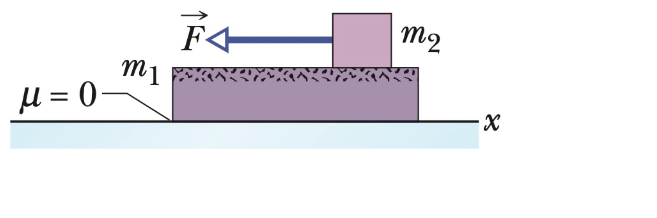The figure below shows two masses m1=5.2 kg and m2=3.4 kg. The lower surface is frictionless but the surface between the blocks has an unknown coefficient of static friction µs. If a horizontal force of magnitude F=59 N acts on the top block and the bottom block moves along with the top block, what is the magnitude of the static friction force which acts between the two blocks? ma Іш U = 0 -X

• ### The figure below shows two masses m =4.1 kg and m2=4.6 kg which are connected by...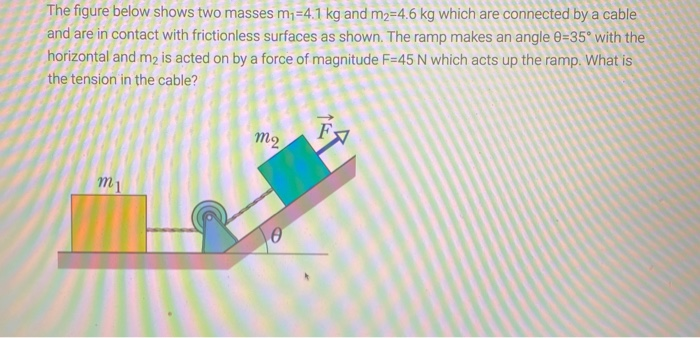The figure below shows two masses m =4.1 kg and m2=4.6 kg which are connected by a cable and are in contact with frictionless surfaces as shown. The ramp makes an angle 9=35° with the horizontal and m2 is acted on by a force of magnitude F=45 N which acts up the ramp. What is the tension in the cable? m2 m1 e

Free Homework App